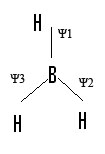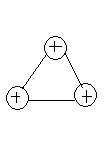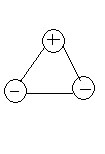# 5.5A: $$BH_3$$

$$\newcommand{\vecs}{\overset { \rightharpoonup} {\mathbf{#1}} }$$ $$\newcommand{\vecd}{\overset{-\!-\!\rightharpoonup}{\vphantom{a}\smash {#1}}}$$$$\newcommand{\id}{\mathrm{id}}$$ $$\newcommand{\Span}{\mathrm{span}}$$ $$\newcommand{\kernel}{\mathrm{null}\,}$$ $$\newcommand{\range}{\mathrm{range}\,}$$ $$\newcommand{\RealPart}{\mathrm{Re}}$$ $$\newcommand{\ImaginaryPart}{\mathrm{Im}}$$ $$\newcommand{\Argument}{\mathrm{Arg}}$$ $$\newcommand{\norm}{\| #1 \|}$$ $$\newcommand{\inner}{\langle #1, #2 \rangle}$$ $$\newcommand{\Span}{\mathrm{span}}$$ $$\newcommand{\id}{\mathrm{id}}$$ $$\newcommand{\Span}{\mathrm{span}}$$ $$\newcommand{\kernel}{\mathrm{null}\,}$$ $$\newcommand{\range}{\mathrm{range}\,}$$ $$\newcommand{\RealPart}{\mathrm{Re}}$$ $$\newcommand{\ImaginaryPart}{\mathrm{Im}}$$ $$\newcommand{\Argument}{\mathrm{Arg}}$$ $$\newcommand{\norm}{\| #1 \|}$$ $$\newcommand{\inner}{\langle #1, #2 \rangle}$$ $$\newcommand{\Span}{\mathrm{span}}$$$$\newcommand{\AA}{\unicode[.8,0]{x212B}}$$Figure 1: Molecule structure of BH3 (Used with permission from Dean.H Johnston)

## Symmetry Labels

 E'' 2 -1 0 -2 1 0 (Rx,Ry) (xz,yz)

FIGURE2: Character table for the the point group D3h

B atom in BH3:

+s-orbital: with the shape of the sphere, its function is x2+y2+z2. Therefore, 2s orbital hasa1' symmetry

+p-orbital: has 3 orbitals , px, py, pz. Therefore, 2pz orbital has a2" symmetry

2px and 2py orbital are degenerate and have e' symmetry

3 Hydrogen atoms in BH3: (Ligand group orbitals)

a. Symmetry labels of LGOs:

-With the symmetry operations of BH3 above, we can determine how many LGO unmoved by creating the following table:D3h E 2C3 3C2 σh 2S3 3σv LGO 3 0 1 3 0 1

-Next, with these values we can apply the following formula to identify the symmetry labels of the Ligan group orbitals

a= 1/h ∑[(N).Xr(R).Xi(R)]

h: the total number of coeficients of symmetry operation

N: the coeficient of the each symmetry operation

Xr(R): the character of the reducible representation corresponding to the R (values that just found in the LGO row

Xi(R): the character of the irreducible representation corresponding to the R (from the character table)

Calculation:

A1'= 1/12 [(1)(3)(1)+(2)(0)(1)+(3)(1)(1)+(1)(3)(1)+(2)(0)(1)+(3)(1)(1)] = 1 A1'

A2'= 1/12 [(1)(3)(1)+(2)(0)(1)+(3)(1)(-1)+(1)(3)(1)+(2)(0)(1)+(3)(1)(-1)]= 0 A2'

E'= 1/12 [(1)(3)(2)+(2)(0)(-1)+(3)(1)(0)+(1)(3)(2)+(2)(0)(-1)+(3)(1)(0)]= 1E'

A1''= 1/12 [(1)(3)(1)+(2)(0)(1)+(3)(1)(1)+(1)(3)(-1)+(2)(0)(-1)+(3)(1)(-1)]= 0 A1''

A2''= 1/12 [(1)(3)(1)+(2)(0)(1)+(3)(1)(-1)+(1)(3)(-1)+(2)(0)(-1)+(3)(1)(1)] = 0 A2''

E''= 1/12 [(1)(3)(2)+(2)(0)(-1)+(3)(1)(0)+(1)(3)(-2)+(2)(0)(1)+(3)(1)(0)] = 0 E ''

Thus, LGO's symmetry labels are a1' + e'

### b. How to determine the shapes of the LGOS?

-LGO's symmetry labels are a1' + e'. There are 3 LGOs that can be made out of these symmetry labels,one LGO is from a1' and two LGOs are from e' due to doubly degenerate. In order to determine the shape of each LGO, we would use the wavefunctions.-Three hydrogens in BH3 are assigned with Ψ1, Ψ2, Ψ3. Now lets look at how each Ψ is affected by the symmetry operations of the D3h and their results are completed in the following table:

 D3h E C3 C23 C2 C2’ C2’’ σh S3 S23 σv σv’ σv’’ Ψ1 Ψ1 Ψ2 Ψ3 Ψ1 Ψ3 Ψ2 Ψ1 Ψ2 Ψ3 Ψ1 Ψ3 Ψ2 a1’ 1 1 1 1 1 1 1 1 1 1 1 1 LGO1 Ψ1 Ψ2 Ψ3 Ψ1 Ψ3 Ψ2 Ψ1 Ψ2 Ψ3 Ψ1 Ψ3 Ψ2

Ψ (a1') = 4Ψ1+4Ψ2+4Ψ3

= 4(Ψ1+Ψ2+Ψ3)

Ψ(a1')= 1/√3 (Ψ1+Ψ2+Ψ3)

-The shape of the LGO1 isD3h E C3 C23 C2 C2’ C2’’ σh S3 S23 σv σv’ σv’’ Ψ1 Ψ1 Ψ2 Ψ3 Ψ1 Ψ3 Ψ2 Ψ1 Ψ2 Ψ3 Ψ1 Ψ3 Ψ2 e’ 2 -1 -1 0 0 0 2 -1 -1 0 0 0 LGO1 2Ψ1 -Ψ2 -Ψ3 0 0 0 2Ψ1 -Ψ2 -Ψ3 0 0 0

Ψ(e') = 4 (Ψ1)-2 (Ψ2)-2 (Ψ3)

= 2[ 2(Ψ1)-Ψ2-Ψ3]

Ψ(e') = 1/ √6 (2 Ψ1-Ψ2-Ψ3)

-The shape of the LGO2 is-Noticed that in the LGO2, we have 1 nodal plane which is the horizonal line between the positive charge and negative charge. Therefore, the LGO3 (doubly degenerate with e') would also 1 nodal plane and its wavefunction would be Ψ(e') = 1/√2 (Ψ2-Ψ3). The shape of the LGO3 is## MO diagram

-As we can see in this diagram, the energy level of 3 LGOs are higher than the 2s orbital and below the 2 p orbital dued to the electronegativy of both Boron and Hydrogen. Hydrogen has higher electronegativity than boron, therefore hydrogen would have lower energy level in the MO diagram.

-In addition, B has 3 electrons in the valence electrons and 3 hydrogens have total 3 electrons. Therefore, the total number of electrons filled in orbitals are 6. With all of the informations above about symmetry labels of B atom and the 3 LGOs, we now construct the MO diagram of BH3. Noticed that, the bonding formation only happens to atoms that have the same symmetry labels. 2s orbital and LGO(1) would contribute 1 electron to give 2 spin pairs electrons at the a1' energy level. 2px and 2py orbitals would bond to the LGO(2) and LGO(3), which give 2 spin pairs electrons at the e' energy level.FIGURE 3: MO diagram for the formation of BH3

-there is a detailed explaination that performed in this video

## References

1. Pople, John A.. Approximate Molecular Orbital Theory (Advanced Chemistry). New York: Mcgraw-Hill (Tx), 1970. Print., Phy Sci Engr Library QD461. P66
2. Noebe, Guillermo (EDT)/, Ronald D. (EDT)/ Abel, and Phillip B. (EDT) Bozzolo. Applied Computational Materials Modeling. Berlin and Heidelberg: Springer Verlag, 2007. Print. Phy Sci Engr Library TA 404.23.A66
3. Housecroft, Catherine, and Alan G. Sharpe. Housecroft Inorganic Chemistry 3e (3rd Edition). 3 ed. Alexandria, VA: Prentice Hall, 2008. 128